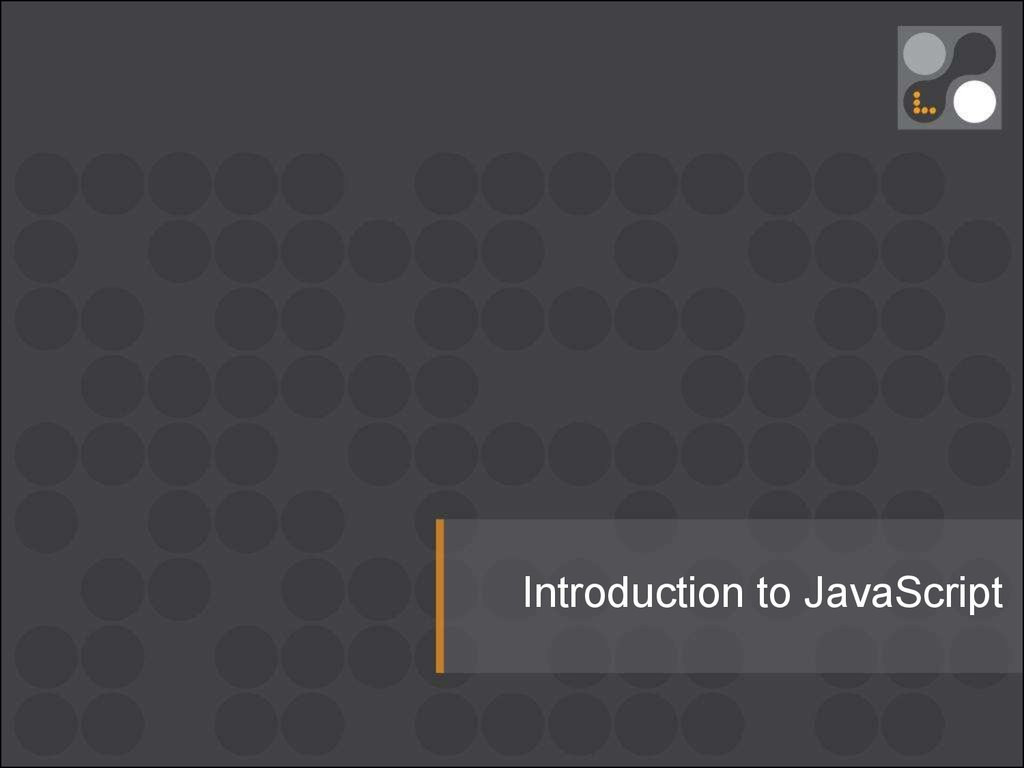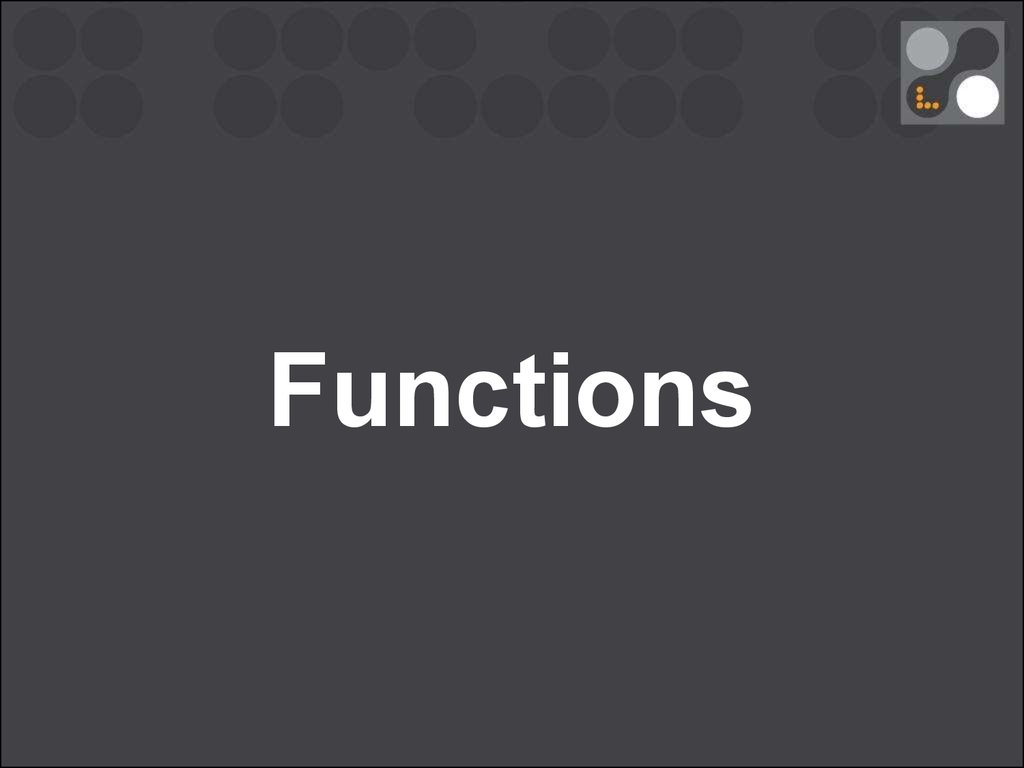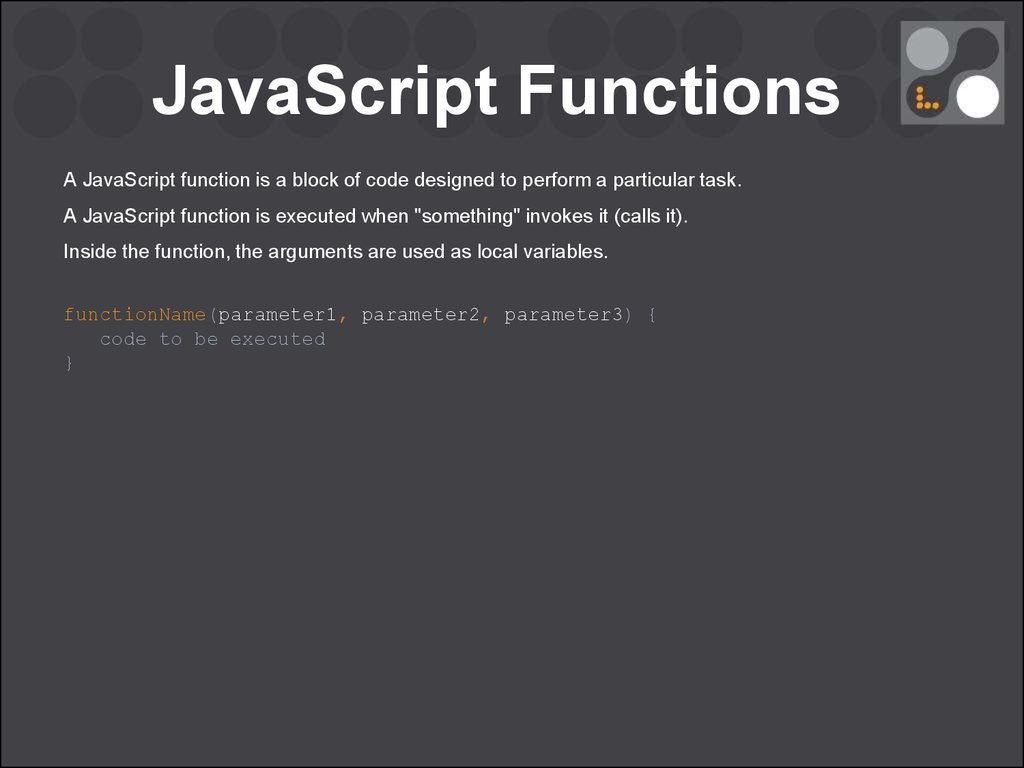# Introduction to JavaScript Functions (part 3)

## 1.

Introduction to JavaScript

Functions

## 3.

JavaScript Functions
A JavaScript function is a block of code designed to perform a particular task.
A JavaScript function is executed when "something" invokes it (calls it).
Inside the function, the arguments are used as local variables.
functionName(parameter1, parameter2, parameter3) {
code to be executed
}

## 4.

JavaScript Functions
A JavaScript function is a block of code designed to perform a particular task.
A JavaScript function is executed when "something" invokes it (calls it).
Inside the function, the arguments are used as local variables.
functionName(parameter1, parameter2, parameter3) {
code to be executed
}
function myFunction(p1, p2) {
return p1 * p2;
}
var x = myFunction(5, 10);
console.log(x);

Functions

## 6.

Functions
Invocation:
When an event occurs (when a user clicks a button)
When it is invoked (called) from JavaScript code
Automatically (self invoked)

## 7.

Functions
Invocation:
When an event occurs (when a user clicks a button)
When it is invoked (called) from JavaScript code
Automatically (self invoked)
Return:
When JavaScript reaches a return statement, the function will stop executing.
The return value is "returned" back to the "caller".

Function Purpose
Information
hiding
Composition
Reuse
Purpose

Function Purpose

## 10.

Function Definition
JavaScript functions are defined with the function keyword.
You can use a function declaration or a function expression.

## 11.

Function Definition
JavaScript functions are defined with the function keyword.
You can use a function declaration or a function expression.
A function expression can be stored in a variable:
var x = function (a, b) {return a * b};

## 12.

Function Definition
JavaScript functions are defined with the function keyword.
You can use a function declaration or a function expression.
A function expression can be stored in a variable:
var x = function (a, b) {return a * b};
After a function expression has been stored in a variable, the variable can be used as a function:
var z = x(4, 3);
The function above is actually an anonymous function (a function without a name).

## 13.

Function Definition
The Function() Constructor:
var myFunction = new Function("a", "b", "return a * b");
// the same
var myFunction = function (a, b) {return a * b};

## 14.

Function Definition
The Function() Constructor: Anti-pattern

## 15.

Function Definition
Try:
// v 1.1
function foo (a, b) {
return a * b;
}
var z = foo (4, 3);
console.log(z);

## 16.

Function Definition
Try:
// v 1.1
function foo (a, b) {
return a * b
}
var z = foo (4, 3);
console.log(z);
// v 1.2
var z = foo (4, 3);
function foo (a, b) {
return a * b;
}
console.log(z);

## 17.

Function Definition
Try:
// v 1.1
// v 1.3
function foo (a, b) {
var x = function (a, b) {return a * b};
return a * b
}
var z = foo (4, 3);
console.log(z);
// v 1.2
var z = foo (4, 3);
function foo (a, b) {
return a * b
}
console.log(z);
var z = x(4, 3);

## 18.

Function Definition
Try:
// v 1.1
// v 1.3
function foo (a, b) {
var x = function (a, b) {return a * b};
return a * b
var z = x(4, 3);
}
var z = foo (4, 3);
// v 1.4
console.log(z);
var z = x(4, 3);
var x = function (a, b) {return a * b};
// v 1.2
var z = foo (4, 3);
function foo (a, b) {
return a * b
}
console.log(z);

## 19.

Function Definition
Try:
// v 1.1
// v 1.3
function foo (a, b) {
var x = function (a, b) {return a * b};
return a * b
var z = x(4, 3);
}
var z = foo (4, 3);
// v 1.4
console.log(z);
var z = x(4, 3);
var x = function (a, b) {return a * b};
// v 1.2
var z = foo (4, 3);
function foo (a, b) {
return a * b
}
console.log(z);
WTF?

## 20.

Hoisting
Hoisting is JavaScript's default behavior of moving declarations to the top of the current scope.
x = 5; // Assign 5 to x
elem = document.getElementById("demo");
elem.innerHTML = x;
var x; // Declare x

## 21.

Hoisting
Hoisting is JavaScript's default behavior of moving declarations to the top of the current scope.
x = 5; // Assign 5 to x
elem = document.getElementById("demo");
elem.innerHTML = x;
var x; // Declare x
JavaScript only hoists declarations, not initializations.
var x = 5; // Initialize x
elem = document.getElementById("demo");
elem.innerHTML = x + " " + y;
var y = 7; // Initialize y

## 22.

Hoisting
Hoisting is JavaScript's default behavior of moving declarations to the top of the current scope.
x = 5; // Assign 5 to x
elem = document.getElementById("demo");
elem.innerHTML = x;
var x; // Declare x
JavaScript only hoists declarations, not initializations.
var x = 5; // Initialize x
elem = document.getElementById("demo");
elem.innerHTML = x + " " + y;
var y = 7; // Initialize y
To avoid bugs, always declare all variables at the beginning of every scope!!!

Hoisting

## 24.

Self-Invoking Functions
You have to add parentheses around the function to indicate that it is a function expression:
(function () {
console.log("Hello!!");
})();
// I will invoke myself

## 25.

Self-Invoking Functions
You have to add parentheses around the function to indicate that it is a function expression:
(function () {
console.log("Hello!!");
})();
WHAT FOR:
-
precompute
-
create scope
// I will invoke myself

## 26.

Function Parameters
Function parameters are the names listed in the function definition.
Function arguments are the real values passed to (and received by) the function.
functionName(parameter1, parameter2, parameter3) {
code to be executed
}

## 27.

Function Parameters
Function parameters are the names listed in the function definition.
Function arguments are the real values passed to (and received by) the function.
functionName(parameter1, parameter2, parameter3) {
code to be executed
}
Parameter Rules:
JavaScript function definitions do not specify data types for parameters.
JavaScript functions do not perform type checking on the passed arguments.
JavaScript functions do not check the number of arguments received.

## 28.

Function Parameters
If a function is called with missing arguments (less than declared), the missing values are set to:
undefined
Assign a default value to the parameter:
function myFunction(x, y) {
y = y || 0;
console.log(x, y);
}

## 29.

Function Parameters
If a function is called with missing arguments (less than declared), the missing values are set to:
undefined
Assign a default value to the parameter:
function myFunction(x, y) {
y = y || 0;
console.log(x, y)
}
If a function is called with too many arguments (more than declared), these arguments cannot be
referred, because they don't have a name. They can only be reached in the arguments object.

## 30.

Arguments Object
The argument object contains an array of the arguments used when the function was called
(invoked).
x = sumAll(1, 123, 500, 115, 44, 88);
function sumAll() {
var i, sum = 0;
for (i = 0; i < arguments.length; i++) {
sum += arguments[i];
}
return sum;
}

## 31.

Arguments Object
The argument object contains an array of the arguments used when the function was called
(invoked).
x = sumAll(1, 123, 500, 115, 44, 88);
function sumAll() {
var i, sum = 0;
for (i = 0; i < arguments.length; i++) {
sum += arguments[i];
}
return sum;
}
Arguments is not really an array. It is an array-like object. arguments has a length property, but it
lacks all of the array methods.

## 32.

Function Invocation
Invoking a function suspends the execution of the current function, passing control and parameters
parameters: this and arguments.

## 33.

Function Invocation
Invoking a function suspends the execution of the current function, passing control and parameters
parameters: this and arguments.
In JavaScript, the thing called this, is the object that "owns" the current code.
*Note that this is not a variable. It is a keyword.

## 34.

Function Invocation
Invoking a function suspends the execution of the current function, passing control and parameters
parameters: this and arguments.
In JavaScript, the thing called this, is the object that "owns" the current code.
*Note that this is not a variable. It is a keyword.
When a function is called without an owner object, the value of this becomes the global object.

## 35.

Invoking a Function as a Method
When a function is stored as a property of an object, we call it a method.
The binding of this to the object happens at invocation time. This very late binding makes functions
that use this highly reusable.
var myObject = {
firstName:"Bilbo",
lastName: "Baggins",
fullName: function () {
return this.firstName + " " + this.lastName;
},
getContex: function () {
return this;
}
}
myObject.fullName();
myObject.getContex();

## 36.

Invoking a Function as a Function
The function does not belong to any object. In a browser the page object is the browser window.
The function automatically becomes a window function.
function myFunction(a, b) {
return a * b;
}
myFunction(10, 2);
window.myFunction(10, 2);
20
// myFunction(10, 2) will return 20
// window.myFunction(10, 2) will also return

## 37.

Invoking a Function as a Function
The function does not belong to any object. In a browser the page object is the browser window.
The function automatically becomes a window function.
function myFunction(a, b) {
return a * b;
}
myFunction(10, 2);
window.myFunction(10, 2);
20
// myFunction(10, 2) will return 20
// window.myFunction(10, 2) will also return
function myFunction() {
return this;
}
myFunction();
// Will return the window object

## 38.

Invoking a Function as a Function
!!! A method cannot employ an inner function to help it to work with object's properties because the
inner function does not share the method's access to the object as its this is bound to the wrong
value.
var add = function (a, b) {
return a + b;
};
var myObject = {
value: 10
}
myObject.double = function () {
var helper = function () {
};
helper();
// Invoke helper as a function.
};
// Invoke double as a method.
myObject.double();
console.log(myObject.value);

## 39.

Invoking a Function as a Function
Fortunately, there is an easy workaround.
var add = function (a, b) {
return a + b;
};
var myObject = {
value: 10
}
myObject.double = function () {
var that = this;
var helper = function () {
};
helper();
// Invoke helper as a function.
};
// Invoke double as a method.
myObject.double();
console.log(myObject.value);

## 40.

Invoking a Function with a
Function Constructor
If a function invocation is preceded with the new keyword, it is a constructor invocation.
It looks like you create a new function, but since JavaScript functions are objects you actually
create a new object:
// This is a function constructor:
function myFunction(arg1, arg2) {
this.firstName = arg1;
this.lastName = arg2;
}
// This creates a new object
var x = new myFunction("Bilbo","Baggins");
x.firstName;

## 41.

Invoking a Function with a
Function Constructor
If a function invocation is preceded with the new keyword, it is a constructor invocation.
It looks like you create a new function, but since JavaScript functions are objects you actually
create a new object:
// This is a function constructor:
function myFunction(arg1, arg2) {
this.firstName = arg1;
this.lastName = arg2;
}
// This creates a new object
var x = new myFunction("Bilbo","Baggins");
x.firstName;
The new prefix also changes the behavior of the return statement.

## 42.

Invoking a Function with a
Function Method
In JavaScript, functions are objects. JavaScript functions have properties and methods.
call() and apply() are predefined JavaScript function methods. Both methods can be used to
invoke a function, and both methods must have the owner object as first parameter. The only
difference is that call() takes the function arguments separately, and apply() takes the function
arguments in an array.
var array = [3, 4];

## 43.

Invoking a Function with a
Function Method
// Create a constructor function called Quo.It makes an object with a status property.
var Quo = function (string) {
this.status = string;
};
// Give all instances of Quo a public method called get_status.
Quo.prototype.get_status = function ( ) {
return this.status;
};
// Make an instance of Quo.
var myQuo = new Quo("confused");
console.log(myQuo.get_status());
// confused

## 44.

Invoking a Function with a
Function Method
// Create a constructor function called Quo.It makes an object with a status property.
var Quo = function (string) {
this.status = string;
};
// Give all instances of Quo a public method called get_status.
Quo.prototype.get_status = function ( ) {
return this.status;
};
// Make an instance of Quo.
var myQuo = new Quo("confused");
console.log(myQuo.get_status());
// confused
// Make an object with a status member.
var statusObject = {
status: 'OK'
};
// statusObject does not inherit from Quo.prototype, but we can invoke the get_status
method on statusObject even though statusObject does not have a get_status method.
var status = Quo.prototype.get_status.apply(statusObject);
// status is 'OK'

## 45.

Good night, folks!